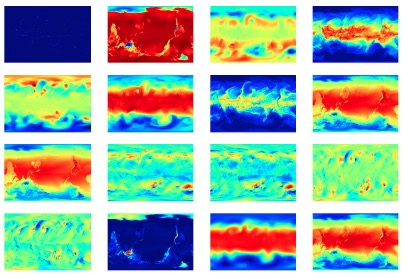# The ExtremeWeather Dataset

• The files are large (62 GB each). Obtain them from Academic Torrents.
• You will need a torrent client for the transfer.

The data is available as one HDF5 file per year, which are formatted like so: “climo_yyyy.h5”, like “climo_1979.h5”.

Each HDF5 file contains two datasets:

• “images”
• “boxes”

Here is a snippet of code to load the datasets with the python library, h5py:

``````import h5py
data_path = "./climo_1979.h5"
h5f = h5py.File(data_path)
images = h5f["images"] # (1460,16,768,1152) numpy array
boxes = h5f["boxes"] # (1460,15,5) numpy array``````

The two variables, "images" and "boxes" are described below:

#### images• a (1460,16,768,1152) array
• 1460 example images (4 per day, 365 days in the year)
• 16 channels in each image corresponding to the following variables
• each channel is 768 x 1152 corresponding to one measurement per 25 square km on earth

#### boxes

• a (1460,15,5) array
• 1460 examples (4 per day, 365 days in the year)
• 15 rows (rows without boxes for that example are filled with -1's)
• each row has 5 elements:

• 4 bounding box coordinates + the class
• ymin, xmin, ymax, xmax, class
• y corresponds to the size 768 dimension and the vertical axis when plotted
• x corresponds to the size 1152 dimension and horizontal when plotted
• classes are types of extreme weather events and go from 0 to 3:

0. Tropical Depression

1. Tropical Cyclone

2. Extratropical Cyclone

3. Atmospheric River

#### Variable Values:

The variables are the 2nd dimension of the "images" dataset in the HDF5 in the following order:

0. PRECT

• Total (convective and large-scale) precipitation rate (liq + ice)

1. PS

• Surface Pressure

2. PSL

• Sea level pressure

3. QREFHT

• Reference height humidity

4. T200

• Temperature at 200 mbar pressure surface

5. T500

• Temperature at 500 mbar pressure surface

6. TMQ

• Total (vertically integrated) precipitatable water

7. TREFHT

• Reference height temperature

8. TS

9. U850

• Zonal wind at 850 mbar pressure surface

10. UBOT

• Lowest model level zonal wind

11. V850

• Meridional wind at 850 mbar pressure surface

12. VBOT

• Lowest model level meridional wind

13. Z100

• Geopotential Z at 100 mbar pressure surface

14. Z200

• Geopotential Z at 200 mbar pressure surface

15. ZBOT

• Lowest model level height